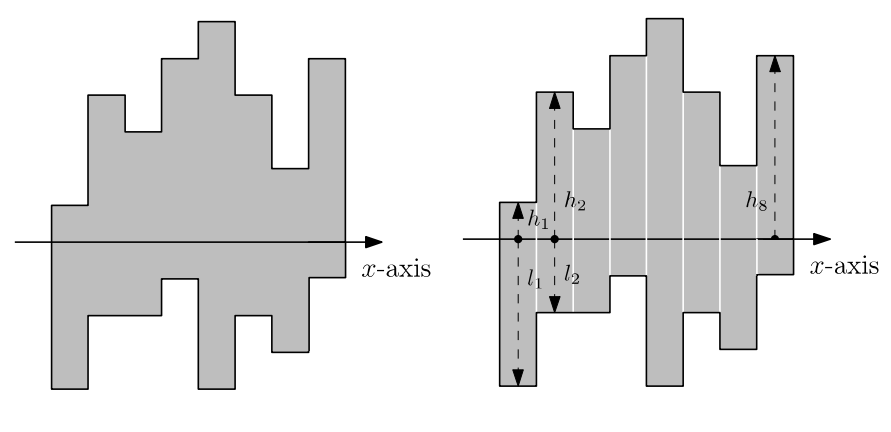시간 제한메모리 제한제출정답맞힌 사람정답 비율
1 초 (추가 시간 없음) 1024 MB2510945.000%

## 문제

ISCO(ICPC Steel Company) is a company that buys steel sheets of a certain shape, cuts them into pieces, and sells them in the industry market. Every steel sheet that ISCO buys has a shape of two histograms of equal width, where one histogram is reflected vertically and soldered to the bottom of the other histogram. This process forms a polygon without holes such that each side is either horizontal or vertical. We call such a polygon a histogon. See the below figure for a histogon.Since the market price of a piece becomes much higher if the steel piece is rectangular, it is desirable to cut a steel sheet into several rectangles. To achieve this, you will use a laser cutter.

In a single operation, the laser cutter can trace either a horizontal segment or a vertical segment through a polygon that touches the border of the polygon at exactly two points, the two endpoints of the segment. After the move, the polygon will be cut into two smaller polygons per the path the laser cutter traced. Note that the laser cutter can only operate on a single polygon in one operation.

The laser cutter is expensive to use, so your task is to find the minimum number of laser cutter operations needed such that after all the operations, all of the resulting polygons are rectangles.

## 입력

The first line of the input contains the number $N$, denoting the width of the histogon. ($1 \le N \le 250\,000$)

The next $N$ lines contain two integers $h_i, l_i$, denoting the height of the first histogram and second histogram, respectively, for the $i$-th column. $h_i$ denotes the height of the $i$-th column of the histogram which is not reflected, and $l_i$ denotes the height of the $i$-th column of the histogram which is reflected. ($1 \le h_i, l_i \le 1\,000\,000$)

## 출력

Print a single integer denoting the minimum operations needed.

## 예제 입력 1

8
1 4
4 2
3 2
5 1
6 4
4 2
2 3
5 1


## 예제 출력 1

7


## 예제 입력 2

5
23 15
23 17
3 22
15 3
5 1


## 예제 출력 2

4